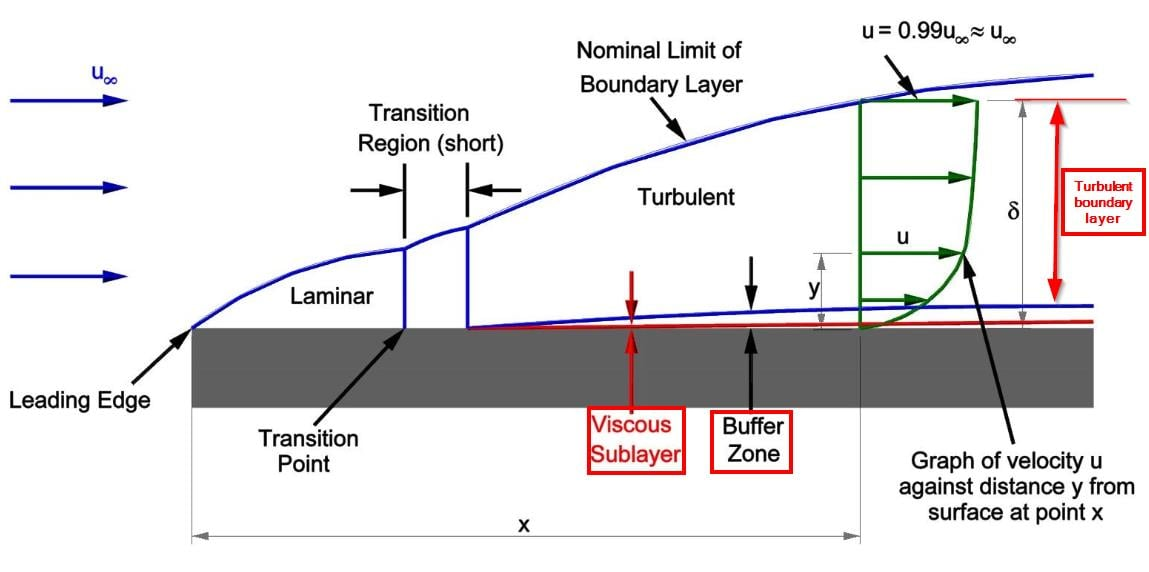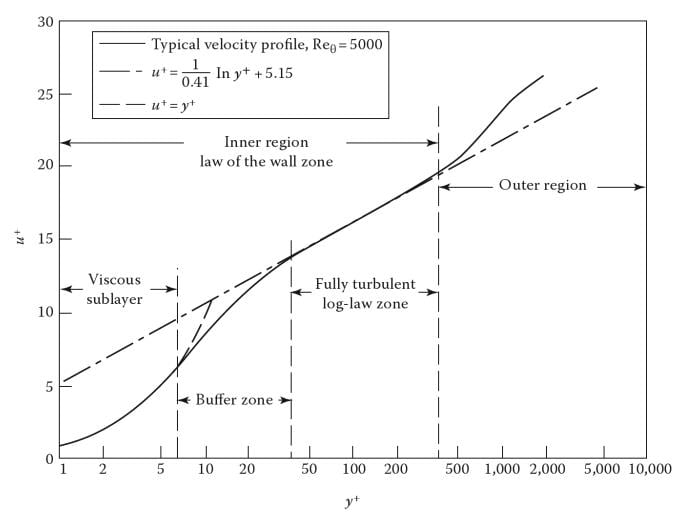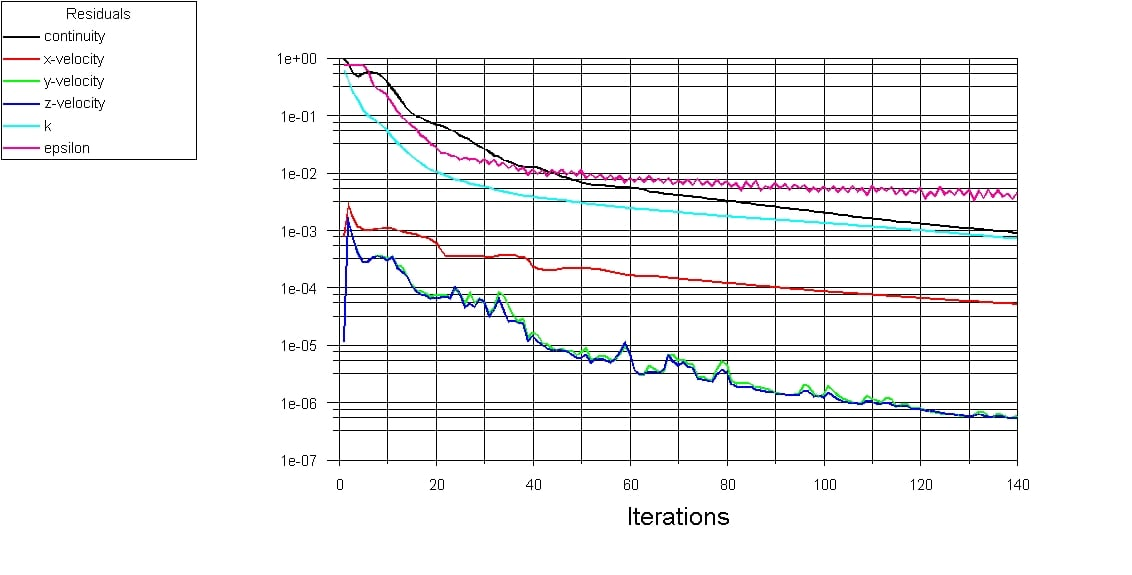## Fluids

•Walaa
Subscriber

What is the the difference between near wall treatment options in Ansys workbench 18.2

•raul.raghav
Subscriber

Boundary layer:

A wall function is needed to accurately predict the flow in the boundary layer. Boundary layer is the thin region near a wall where the velocity gradient in the direction normal to the wall is high (the velocity goes from zero at the wall to ~ the mainstream velocity at a certain distance away from the wall). The boundary layer exists for all flows, let it be laminar, transitional or turbulent. A laminar flow would have a laminar boundary layer, which is straightforward. On the other hand, a turbulent boundary layer would have a very thin viscous sub-layer next to wall, a transition layer (buffer zone) and the turbulent boundary layer (log-layer). The turbulent boundary layer on a flat plate and the velocity profile across a turbulent boundary layer is shown in the image below.Why do we need a wall function?

From CFD standpoint, it is essential that we create a mesh/grid which can accurately predict the velocity gradient across boundary layer. For turbulent flows, we would ideally want the first cell from the wall to lie within the very thin viscous sub-layer. Though this might be possible for certain flow scenarios, this criteria cannot be fulfilled for complex flows in complicated geometries as it would require a very fine mesh resolution near the wall which would extensively increase the time required for solving the problem. To deal with this requirement, a wall function is introduced which allows the use of a "relatively" larger mesh near the vicinity of the wall. Now depending on the region of the boundary layer you want to capture, you would need the appropriate mesh and appropriate wall function. Another important parameter when it comes to wall functions is y-plus (y+). y+ is the non-dimensional distance from the wall to the first node from the wall. The different regions of the turbulent boundary layer based on y+ would be: laminar sub-layer (y+ <5); transition or buffer layer (5 < y+ <30); and turbulent or log-layer (y+ >30). The velocity is linear in the viscous sub-layer while its logrithmic for the log-layer (see image below). One important point is that the first cell adjacent to the wall should not lie in the buffer zone, i.e., y+ should not be between 5 and 30 (Not good: 5 > y+ > 30).Near Wall Treatment for k-e turbulence model in Ansys Fluent:

Standard wall function:
If the first cell cannot be placed within the viscous sub-layer and it lies in the log-layer region, standard wall functions can be employed. "They provide reasonably accurate predictions for the majority of high-Reynolds-number, wall-bounded flows." The y+ value should be between 30 and 300 (valid: 30 > y+ >300).

Non-equilibrium wall function:
Under severe pressure gradient and strong non-equilibrium flows, the standard wall functions do not predict the flow accurately. So the non-equilibrium wall function provides a better prediction. "Because of the capability to partly account for the effects of pressure gradients and departure from equilibrium, the non-equilibrium wall functions are recommended for use in complex flows involving separation, reattachment, and impingement where the mean flow and turbulence are subjected to severe pressure gradients and change rapidly." The y+ value should be between 30 and 300, just like the standard wall function (valid: 30 > y+ >300).

Enhanced wall treatment:
When the viscous sub-layer needs to be captured in cases like transitional flow, separation, heat transfer, frictional drag prediction etc., the first cell has to be located within the viscous sub-layer and the y+ should be less than 1 (valid: y+ < 1). For complicated geometries, the y+ can go upto 5 (valid: y+ < 5).

1. A very good blog post explaining the above concepts with respect to the mesh near the wall, turbulence model and the wall function is provided below:

Resolving the boundary layer

Estimating the First Cell Height for correct Y+

•Walaa
Subscriber

Thanks a bunch Raul.

•José Mantovani
Subscriber

Just to complement, the interest in obtaining a mesh with a satisfactory Y + number is due to the fact that in order to calculate the drag coefficient, that in a certain way, the experimental result coincides with the numerical result, this approach is necessary. The calculation is much more accurate (to calculate the wall shear) from a refined cell near the wall than by the cell center value, due to the high gradients. However, in an external flow, for example, around a wing as the angle of attack increases this approach is no longer useful due to the detachment of the boundary layer.

Hugs,

Mantovani.

•Raef.Kobeissi
Subscriber

Also to add, there is an approximative method to calculate the thickness of the inflation layer to achieve a desired Y+ value:

https://www.cfd-online.com/Wiki/Dimensionless_wall_distance_(y_plus)

Also this online calculator:

https://www.raefkobeissi.com/yplus/yplus.aspx

•salah
Subscriber

Hi friends

I am a first year doctoral student at the university. currently I imply CFD modeling in fluent. I spent a year in the mesh, could you help me choose the best mesh for (y plus) and (dy first cell no mesh) for a turbulent simulation with number of Re = 6000 to 100000 to calculate the constant (of the logarithmic profile of the law of log.

Vx / (V *) = 2.5 Ln (y / k) + B.

with artificial roughness effect

1. Please explain to me how y + was chosen, how the turbulence model was chosen (k epsilon, k-w, scalable or improved RNG or standard ...)

2. Can I use the same mesh to calculate the shear stress and the logarithmic profile and the constant B

•DrAmine
Ansys Employee

1/Better to open a new thread

2/Use a fine mesh with yplus around 1 to 5 if using two equations turbulence models

3/New roughness models requires even finer mesh

•salah
Subscriber

thank you very much Amin

but what do i use? the k epsilon or k omega model.

-if y + between 1 and 5 why they said that y + greater than 30 for the logarithmic

profile is that I am looking for.

- I have another question for high reynolds numbers 55000, my simulation diverges with k epsilon enhanced•Dilini
Subscriber
Hello,nIn my case I am using SST model. My first cell height is an extremely small value (around 5*10-7 m) When I use this I get the floating point error. When I use a coarse mesh and proceed my simulation proceeds without any issue.nSo the problem is with my mesh. But I am not sure how to fix it. I use inflation and make my first cell height the above value.nPlease any help would be highly appreciated. Been stuck for days in this now.nThank you.nRegardsnDilinin